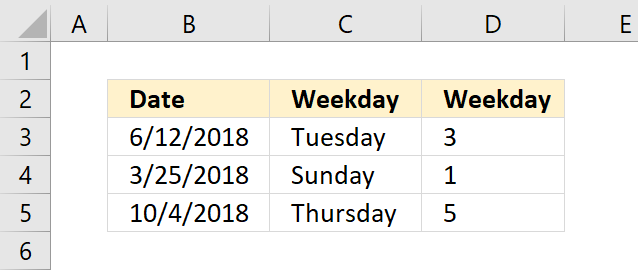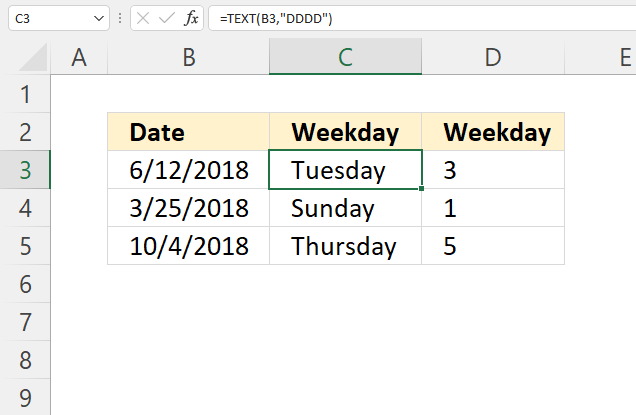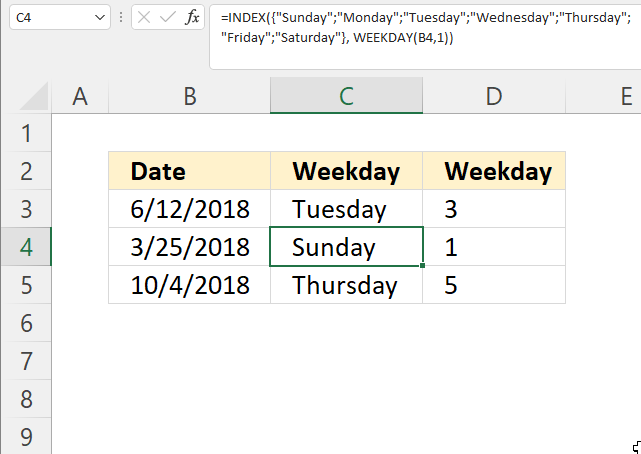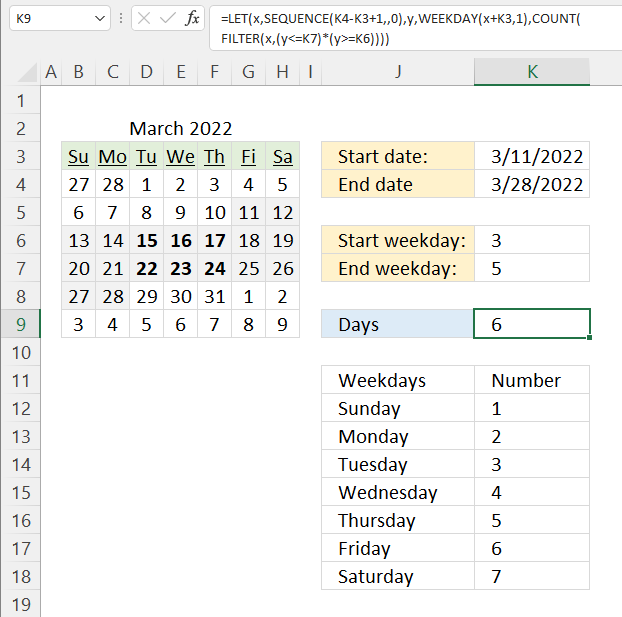Author: Oscar Cronquist Article last updated on February 07, 2023The WEEKDAY function converts a date to a weekday number from 1 to 7. You can customize the function so the week starts with any weekday.

A weekday is a day of the week except for Saturday and Sunday, however, the WEEKDAY function returns Saturdays and Sundays as well.

## 1. WEEKDAY Function Syntax

WEEKDAY(serial_number,[return_type])

## 2. WEEKDAY Function Arguments

 serial_number Required. The Excel date value you want to extract the WEEKDAY number from. return_type Optional. Determines the type of return value, see table below.

 Return_type Number returned 1 or omitted Numbers 1 to 7 for Sunday to Saturday. 2 Numbers 1 to 7 for Monday to Sunday. 3 Numbers 0 to 6 for Monday to Sunday. 11 Numbers 1 to 7 for Monday to Sunday. 12 Numbers 1 to 7 for Tuesday to Monday. 13 Numbers 1 to 7 for Wednesday to Tuesday. 14 Numbers 1 to 7 for Thursday to Wednesday. 15 Numbers 1 to 7 for Friday to Thursday . 16 Numbers 1 to 7 for Saturday to Friday . 17 Numbers 1 to 7 for Sunday to Saturday.

## 4. WEEKDAY function exampleThe WEEKDAY function shown in cell D3 in the image above calculates a number based on a date. The number represents a given weekday based on the provided value in the second argument.

The formula below has no second argument, it defaults to 1 meaning the week begins with a Sunday.

Formula in cell D3:

=WEEKDAY(B3)

## 5. WEEKDAY function not working

• Check that you are using a valid Excel date.
• Check the number of arguments.

## 6. WEEKDAY function - show nameFormula in cell C3:

=TEXT(B3, "DDDD")

#### Step 1 - TEXT function

The TEXT function lets you format values.

TEXT(value, format_text)

value - The string you want to format. You can use a cell reference here or use a text string.

format_text - Formatting code allowing you to change the way, for example, a date or a number is displayed to the Excel user.

#### Step 2 - Populate arguments

The TEXT function has two arguments.

value - B3

format_text - "dddd"

"dddd" returns the weekday. Lower and upper case letters make no difference.

#### Step 3 - Evaluate TEXT function

TEXT(B3, "dddd")

becomes

TEXT(43263, "dddd")

and returns "Tuesday".Formula in cell C4:

=INDEX({"Sunday";"Monday";"Tuesday";"Wednesday";"Thursday";"Friday";"Saturday"}, WEEKDAY(B4,1))

### Explaining formula

#### Step 1 - Calculate number representing a weekday

WEEKDAY(B4,1)

becomes

WEEKDAY(43184, 1)

and returns 1.

#### Step 2 - Return weekday name based on position in array

The INDEX function returns a value in a cell range or array based on a row and column number (optional).

INDEX(array[row_num][column_num], [area_num])

INDEX({"Sunday";"Monday";"Tuesday";"Wednesday";"Thursday";"Friday";"Saturday"}, WEEKDAY(B4,1))

becomes

INDEX({"Sunday";"Monday";"Tuesday";"Wednesday";"Thursday";"Friday";"Saturday"}, 1)

and returns "Sunday". "Sunday" is the first value in the array.

## 7. Count days between two given weekdays and two datesThe image above shows an Excel 365 formula that counts days between two dates and also if they are between two given weekdays.

The start date is specified in cell K3, end date is in cell K4. The start weekday is 3 which represents Tuesday, the table in J11:K18 shows the numbers and corresponding weekday names. The end weekday is 5 which represents Thursday.

The calendar in cell range B4:H9 shows which days (bolded) meet the criteria. Cell K9 displays the result, there are six bolded days in the calendar.

Excel 365 dynamic array formula in cell K6:

=LET(x,SEQUENCE(K4-K3+1,,0),y,WEEKDAY(x+K3,1),COUNT(FILTER(x,(y<=K7)*(y>=K6))))

### Explaining formula

COUNT(FILTER(SEQUENCE(K4-K3+1,,0),(WEEKDAY(SEQUENCE(K4-K3+1,,0)+K3,1)<=K7)*(WEEKDAY(SEQUENCE(K4-K3+1,,0)+K3,1)>=K6)))

#### Step 1 - Calculate the number of days and add one

The minus and plus signs let you perform arithmetic operations in an Excel formula

K4-K3+1

44648 - 44631 +1

equals 18.

#### Step 2 - Create a sequence of numbers from 0 to 18

The SEQUENCE function creates a list of sequential numbers to a cell range or array. It is located in the Math and trigonometry category and is only available to Excel 365 subscribers.

SEQUENCE(rows, [columns], [start], [step])

SEQUENCE(K4-K3+1,,0)

becomes

SEQUENCE(18,,0)

and returns

{0; 1; 2; 3; 4; 5; 6; 7; 8; 9; 10; 11; 12; 13; 14; 15; 16; 17}.

#### Step 3 - Add date to sequence of numbers

The plus sign lets you perform add numbers in an Excel formula.

SEQUENCE(K4-K3+1,,0)+K3

becomes

{0; 1; 2; 3; 4; 5; 6; 7; 8; 9; 10; 11; 12; 13; 14; 15; 16; 17} + 44631

and returns

{44631; 44632; 44633; 44634; 44635; 44636; 44637; 44638; 44639; 44640; 44641; 44642; 44643; 44644; 44645; 44646; 44647; 44648}.

#### Step 4 - Calculate weekday

WEEKDAY(SEQUENCE(K4-K3+1,,0)+K3,1)

becomes

WEEKDAY({44631; 44632; 44633; 44634; 44635; 44636; 44637; 44638; 44639; 44640; 44641; 44642; 44643; 44644; 44645; 44646; 44647; 44648},1)

and returns

{6; 7; 1; 2; 3; 4; 5; 6; 7; 1; 2; 3; 4; 5; 6; 7; 1; 2}.

#### Step 5 - Check if number is less than seven

The less than and larger than characters let you check if a value is smaller or larger than a condition, the result is a boolean value TRUE or FALSE. The equal sign and the less than character combined compare if values are equal or smaller than a condition.

WEEKDAY(SEQUENCE(K4-K3+1,,0)+K3,1)<=K7

becomes

{6; 7; 1; 2; 3; 4; 5; 6; 7; 1; 2; 3; 4; 5; 6; 7; 1; 2}<=5

and returns

{FALSE; FALSE; TRUE; TRUE; TRUE; TRUE; TRUE; FALSE; FALSE; TRUE; TRUE; TRUE; TRUE; TRUE; FALSE; FALSE; TRUE; TRUE}.

#### Step 6 - Check if weekday is larger than one

WEEKDAY(SEQUENCE(K4-K3+1,,0)+K3,1)>=K6

becomes

{6; 7; 1; 2; 3; 4; 5; 6; 7; 1; 2; 3; 4; 5; 6; 7; 1; 2}>=3

and returns

{TRUE; TRUE; FALSE; FALSE; TRUE; TRUE; TRUE; TRUE; TRUE; FALSE; FALSE; TRUE; TRUE; TRUE; TRUE; TRUE; FALSE; FALSE}.

#### Step 7 - Multiply arrays

The asterisk character lets you multiple values in an Excel formula. Multiplying boolean values always return their numerical equivalents.

TRUE -> 1

FALSE -> 0 (zero)

(WEEKDAY(SEQUENCE(K4-K3+1,,0)+K3,1)<7)*(WEEKDAY(SEQUENCE(K4-K3+1,,0)+K3,1)>1)

becomes

{FALSE; FALSE; TRUE; TRUE; TRUE; TRUE; TRUE; FALSE; FALSE; TRUE; TRUE; TRUE; TRUE; TRUE; FALSE; FALSE; TRUE; TRUE}*{TRUE; TRUE; FALSE; FALSE; TRUE; TRUE; TRUE; TRUE; TRUE; FALSE; FALSE; TRUE; TRUE; TRUE; TRUE; TRUE; FALSE; FALSE}

and returns

{0; 0; 0; 0; 1; 1; 1; 0; 0; 0; 0; 1; 1; 1; 0; 0; 0; 0}.

#### Step 8 - Filter numbers based on condition

The FILTER function is a new function available to Excel 365 subscribers. It lets you extract values based on a condition or criteria.

FILTER(arrayinclude, [if_empty])

FILTER(SEQUENCE(K4-K3+1,,0),(WEEKDAY(SEQUENCE(K4-K3+1,,0)+K3,1)<7)*(WEEKDAY(SEQUENCE(K4-K3+1,,0)+K3,1)>1))

becomes

FILTER({0; 1; 2; 3; 4; 5; 6; 7; 8; 9; 10; 11; 12; 13; 14; 15; 16; 17},{0; 0; 0; 0; 1; 1; 1; 0; 0; 0; 0; 1; 1; 1; 0; 0; 0; 0})

and returns

{4; 5; 6; 11; 12; 13}.

#### Step 9 - Count numbers in the array

The COUNT function counts all numbers in a cell range or array.

COUNT(value1, [value2], ...)

COUNT(FILTER(SEQUENCE(K4-K3+1,,0),(WEEKDAY(SEQUENCE(K4-K3+1,,0)+K3,1)<7)*(WEEKDAY(SEQUENCE(K4-K3+1,,0)+K3,1)>1)))

becomes

COUNT({4; 5; 6; 11; 12; 13})

and returns 6. There are six numbers in the array.

#### Step 10 - Shorten formula

The LET function allows you to name intermediate calculation results which can shorten formulas considerably and improve performance.

LET(name1name_value1calculation_or_name2, [name_value2calculation_or_name3...])

LET(x, SEQUENCE(K4-K3+1, , 0), y, WEEKDAY(x+K3, 1), COUNT(FILTER(x, (y<7)*(y>1))))

x - SEQUENCE(K4-K3+1,,0)

y - WEEKDAY(x+K3,1)